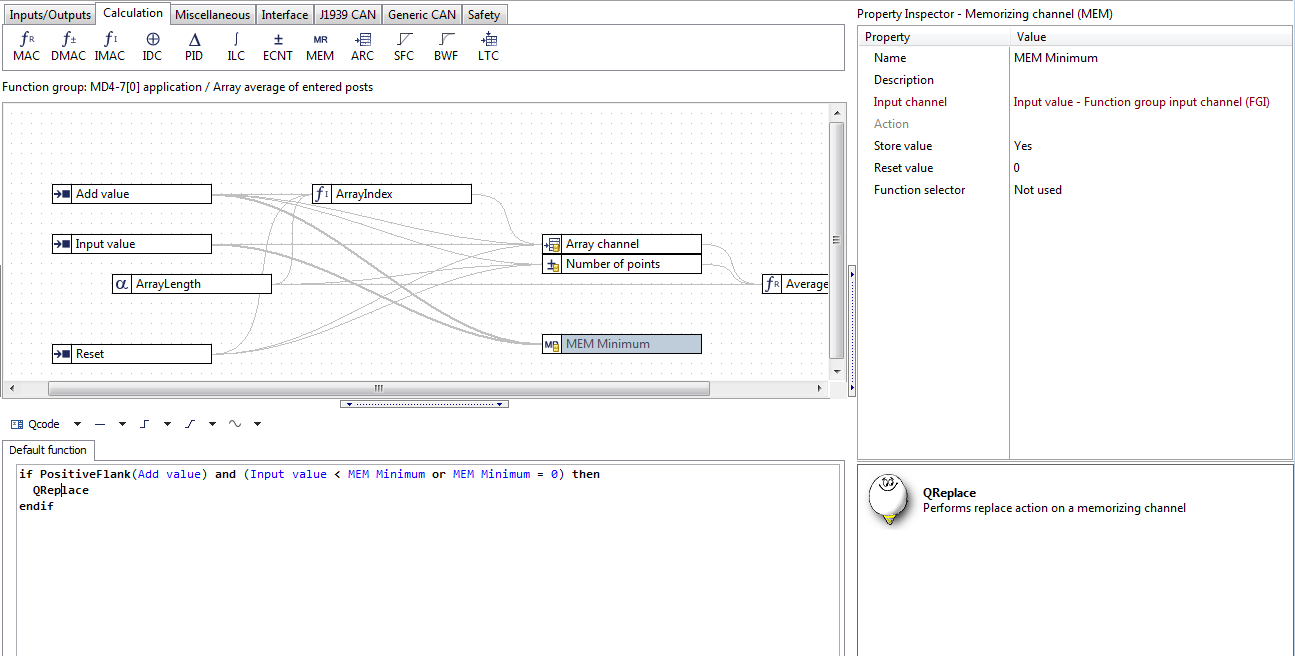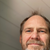0

# Array problem

Tuomas 7 years ago in IQANdesign updated by Pierre Fagrell 3 years ago

Example: I have array what size is 100 and If I have only 60 measurements there, How I get minimum and average values from these measurements?

ArrayIndexOfMin and ArrayAverage calculates all array 100 values even these empty 40 records and then I get wrong calculations.

How do I get minimum and average values only from records what contains measurements bigger than 0?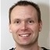+1

Hi,

That is an interesting question. My immediate thought was to start calculating totals using a separate channel, but a more efficient way is to just keep track of the number of posts you have entered, and use these to scale the result from the ArrayAaverage() function.

if Number of points > 0 then

Result := (ArrayAverage(Array channel)/Number of points) *ArrayLength

endif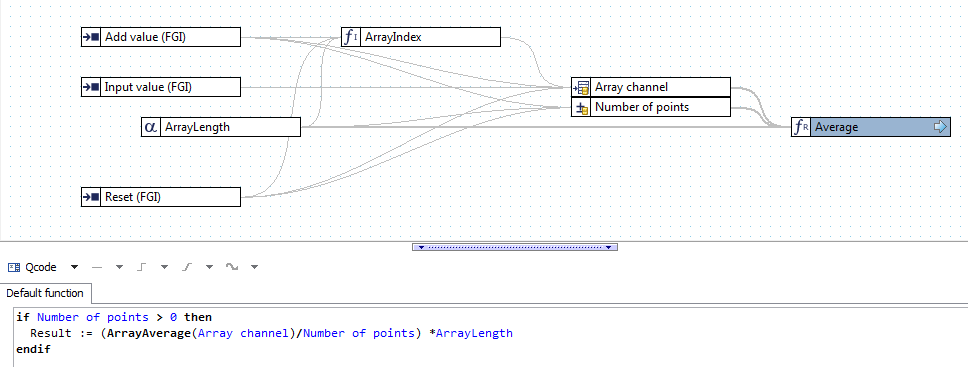Attached is a project file that impelments this idea: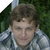+1

This is a useful example of how to use the array channel beyond what is in the manual. Perhaps it could be added to the solutions library.

Is it still the most efficient way or are there any improvements in IQANdesign 6 that could be utilized?Thanks, I agree that it could be nice to have in the solutions library to illustrate the use of arrays.

The only small performance improvement I can think of is to make the integer parameter for array size a constant channel. Only saves that one channel from being calculated every cycle, and has the drawback that it has to be public when it is only needed in this FG.+1

By building on your example and chaining three array channels I have made a log of time series data similar to RRDtool.

I find this kind of graphs useful with short timebases when building prototypes or long timebases and storage channels for logging production parameters over hours/days/years (be careful not to store short timebases to flash).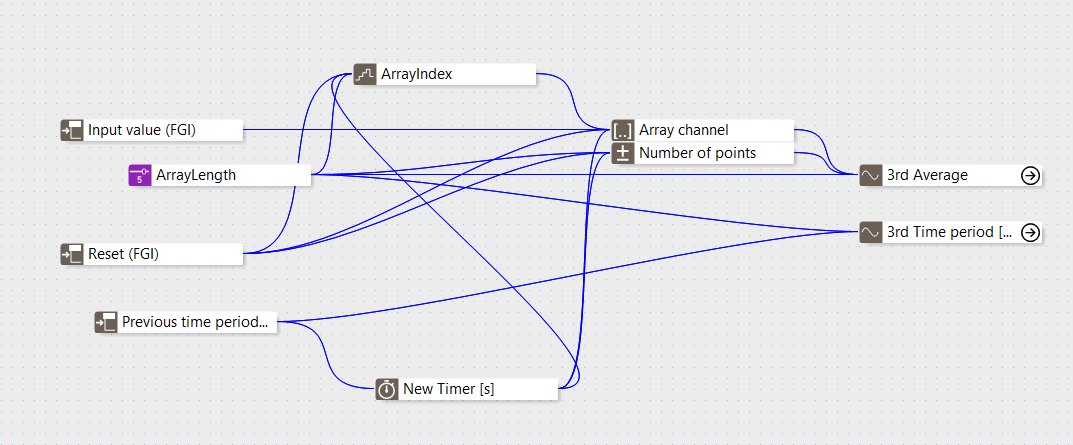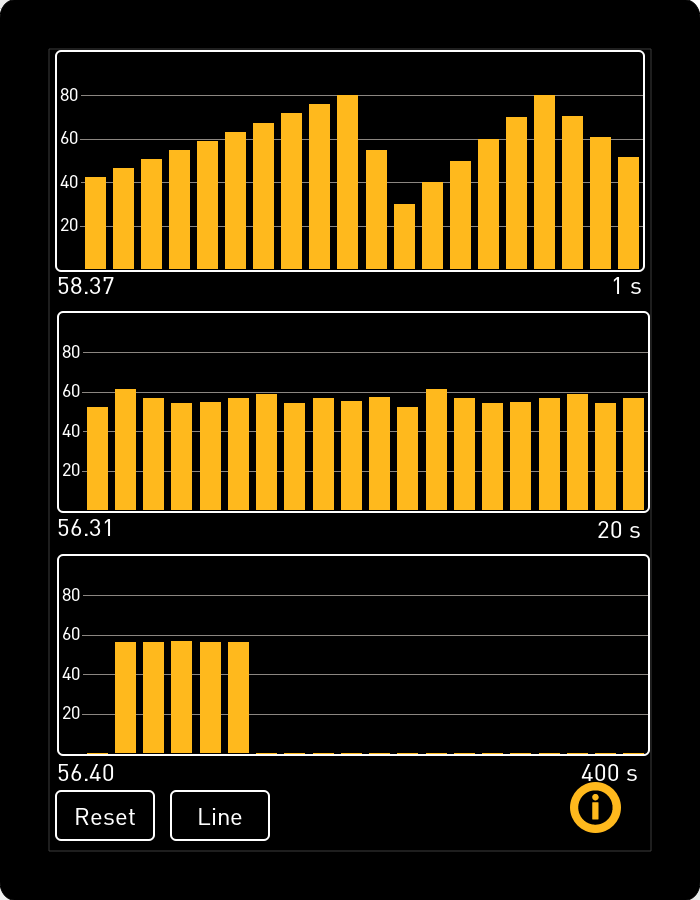Average.idsxHow about to find minimum value?

Now its find always 0 for minimum (if you have some empty records in array) even real minimum measurement is more than it.

Should I save all measurements to MEM function and compare each measure to MEM value?

If measurement is smaller than MEM value -> reset MEM with new value.

Can you improve Array function to calculate only values greater than 0?

Or make it on option, so you don't have to do extra functions like these examples?+1

Yes, using a MEM channel would be the way to find the minimum. Something like this:

`if PositiveFlank(Add value) and (Input value < MEM Minimum or MEM Minimum = 0) then`

`QReplace`

`endif`